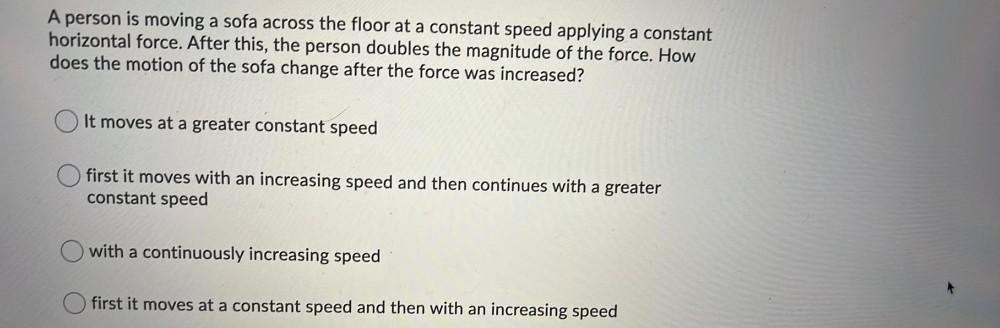Question:

# A person is moving a sofa across the floor at a constant speed applying a constant horizontal force. After this, the person doubA person is moving a sofa across the floor at a constant speed applying a constant horizontal force. After this, the person doubles the magnitude of the force. How does the motion of the sofa change after the force was increased? It moves at a greater constant speed first it moves with an increasing speed and then continues with a greater constant speed O with a continuously increasing speed first it moves at a constant speed and then with an increasing speed